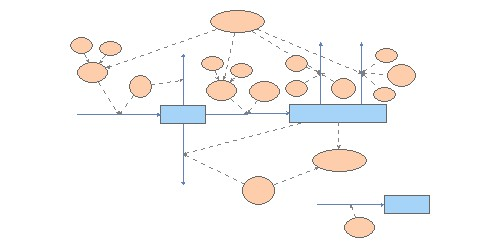Clone of energy SFG mussel with allometriesIndividual physiological responses were converted into energy equivalents and used in the balanced energy equation:

C = P + R + U + F

where:
C = total consumption of food energy;
P = Production of both somatic and reproductive tissue
R = energy expenditure in respiration
U = energy loss in excretion
F = energy loss in feaces and pseudofeaces

The absorbed energy, A, is the product of consumption, C, and the absorption efficiency of energy from the food. Production may be expressed as:

P = A - (R + U)

Production can be referred as "Scope for growth" or the energy that the animal can use for growing.

Calculation of C, A, R and U (J/g h) is as follows:

C = CR (L/g h) * POM (mg/L) * 23 J/mg ash-free dry weight;
A = C * AE
R = VO2 (mlO2 / g h) * 20.33 J/ml O2
U = mg NH4/ g h * 19.4 J/ mg NH4

CR is the volume of water
cleared of particles per gram mussel per hour and was calculated according to the expression: